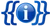136Template documentation

This template is used to display the current number of member states which recognize a particular state. To update the number, month, year, or name produced by this template, you must modify the corresponding subtemplate.

### Usage

The first parameter corresponds to a subtemplate of this template, e.g., `Pal` for {{Numrec/Pal}}. The second and third parameter inserts wikilinked text before and after the number. Other optional parameters are:

• `asof = S` to turn on the "As of" prefix (start)
• `asof = E` to turn on the "as of" suffix (end)
• `alt = foo` to change the "As of" prefix to "foo"
• `alt2 = bar` to add "bar" after the "As of" date and before the wikilinked text
• `link = N` to turn off linking
• `pcent = total number` to add a percentage out of "total number". If pcent=UN then the total number of UN member states is used.
• `N = number` outputs "Number of recognizers + N". This is useful in situations where non-UN member states are to be included in the total.
• `W = Y` outputs number of states who have withdrawn recognition as opposed to the number of recognizers.

### Examples

• `{{Numrec|Pal|the|countries}}`the 136 countries
• `{{Numrec|Pal|the|countries|link=N}}` → the 136 countries
• `{{Numrec|Pal|although|of the countries|link=N|pcent=193}}` → although 70.5% of the countries
• `{{Numrec|Pal|a total of|countries ...|link=N|asof=S}}` → As of 14 September 2015, a total of 136 countries ...
• `{{Numrec|Pal|a total of|countries ...|link=N|asof=S|alt=as of }}` → as of 14 September 2015, a total of 136 countries ...
• `{{Numrec|Pal|a total of|countries ...|link=N|asof=E}}` → a total of 136 countries ... as of 14 September 2015
• `{{Numrec|Pal|a total of|countries ...|link=N|asof=E|alt=, by }}` → a total of 136 countries ... , by 14 September 2015